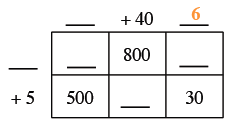### Home > CC1 > Chapter 4 > Lesson 4.2.1 > Problem4-50

4-50.

Copy and complete the generic rectangle below. What multiplication problem does it represent and what is the product? Homework Help ✎

 _____ $+40$ _____ _____ _____ $800$ _____ $+5$ $500$ _____ $30$

For generic rectangles, remember that the product it represents is simply the sum of the areas within the rectangle.

To get started, here is one of the missing numbers. Can you see why it is correct?